# Java Program to Check Armstrong Number

In this tutorial you will learn about the Java Program to Check Armstrong Number and its application with practical example.

In this tutorial, we will learn to create a Java program that will Check Armstrong Number   using Java programming.

## Prerequisites

Before starting with this tutorial we assume that you are best aware of the following Java programming topics:

• Java Operators.
• Basic Input and Output
• Class and Object.
• Basic Java programming.
• Loops.
• If-else statement.

## What Is Armstrong Number?

A number is called An Armstrong  is a number in which the sum of the cube of the individual digits in number  is equal to the number itself. For example 0, 1, 153, 370, 371 and 407 are the Armstrong numbers. Let’s try to understand why 153 is an Armstrong number.

407 = ((4*4*4)+(0*0*0)+(7*7*7) )

407 =( 64 + 0 + 343)

407 = 407 (So this is an Armstrong number.)

Similarly, Let’s take number 370 and understand why 370 is an Armstrong number.

370=(3*3*3+7*7*7+0*0*0)

370=(27+343+0)

370=370.(So this is a Armstrong number.)

## Java Program to Check Armstrong Number

In this program we will find whether a given three digit number is Armstrong number or not using while loop. We would first declared and initialized the required variables. Next, we would prompt user to input a three digit number. Later we will find given no is Armstrong number or not.

## Output

Armstrong Number..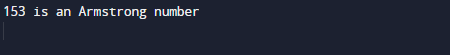Not a Armstrong Number.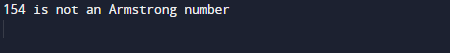In the above program, we have first declared and initialized a set variables required in the program.

• no = take given number by user.
• numb= to copy original no.
• temp= for holding remainder
• armno= for result.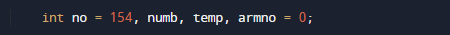Here we created a program in which we find a three digit number is a Armstrong number or not. First we copy the original no to numb variable and then with the help of while loop iterate loop until we reach the last value or given no.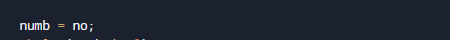And in loop we break the number’s  last digit and multiply this number three time itself and store in armno variable and after that we break  numb to two digit no with (/) operator and copy the new two digit number in our armno variable and repeat this process to last number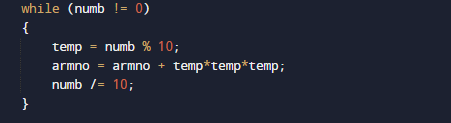then we compare the given number and armno is equal it to the given no if they are equal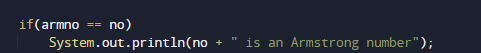then the number is Armstrong number else the given number is not a Armstrong number.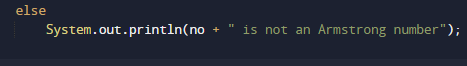In this tutorial we have learn about the Java Program to Check Armstrong Number and its application with practical example. I hope you will like this tutorial.